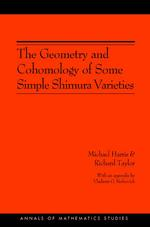# The Geometry and Cohomology of Some Simple Shimura Varieties. (AM-151)

Michael Harris
Richard Taylor
With an appendix by Vladimir G. Berkovich
Pages: 288
https://www.jstor.org/stable/j.ctt1287kfd

1. Front Matter
(pp. i-vi)
(pp. vii-viii)
3. Introduction
(pp. 1-14)

This book has twin aims. On the one hand we prove the local Langlands conjecture forGLnover ap-adic field. On the other hand in many cases we are able to identify the action of the decomposition group at a prime of bad reduction on thel-adic cohomology of the “simple” Shimura varieties studied by Kottwitz in [Ko4]. These two problems go hand in hand.

The local Langlands conjecture is one of those hydra-like conjectures which seems to grow as it gets proved. However the generally accepted formulation seems to be the following (see [He2]). LetKbe a...

4. Acknowledgements
(pp. 15-16)
5. Chapter I Preliminaries
(pp. 17-58)

In this chapter we will establish some notation, recall some standard facts and prove a couple of technical lemmas. The reader may like to read only section 1.7 (which establishes some very important assumptions which are particular to this book) and simply refer back to the rest of this chapter as the need arises.

In this section we will introduce some notation which we will use throughout this book.

We willlet p and I denote rational primes.We will ojten assume that$I \ne p$. ln particular from the middle of section III.4 onwards this will always be the case.Let${Z_{(p)}}$...

6. Chapter II Barsotti-Tate groups
(pp. 59-88)

For the definition of a Barsotti-Tate group over a schemeSwe refer the reader to section 2 of chapter I of [Me]. Suppose thatSis aOKscheme, then by a Barsotti-TateOK-moduleH/Swe shall mean a Barsotti-Tate groupH/Stogether with an embedding${O_K} \to End(H).$(We remark that ring morphisms are assumed to send the multiplicative identity to itself.) We calI a Barsotti-Tate${O_K}$-moduleHind-etale if the underlying BarsottiTate group is ind-etale (see example 3.7 of chapter l of [Me]). There is an equivalence of categories between ind-etale Barsotti-Tate${O_K}$-modules and finite, torsion free lisse...

7. Chapter III Some simple Shimura varieties
(pp. 89-120)

In this section we shall introduce some Shimura varieties which will be the main object of study in this book. Our class is a sub-class of the class singled out by Kottwitz in [Ko4]. Our class combines the simple group theoretic properties of Kottwitz’s examples with some additional simple geometric properties.

We will use without comment notation established in section 1.7.

Let us recall a lemma about abelian schemes.

Lemma 111.1.1Let L be a totally real field, I be a rational prime, S an L-scheme, A/S an abelian scheme and i:L$\to En{d^0}$(A) an embedding. Suppose that for...

8. Chapter IV Igusa varieties
(pp. 121-148)

In our setting there seem to be two natural analogues of the familiar Igusa curves in the theory of elliptic modular curves. We will call these Igusa varieties of the first and second kind. When we refer to these Igusa varieties we will refer only to the analogue of the ordinary locus on the usual Igusa curves. We have not looked at the question of whether our Igusa varieties admit natural smooth compactifications, although we feel this is a natural and interesting questiono ln the case of elliptic modular curves, the Weil pairing on thep-divisible group of an elliptic...

9. Chapter V Counting Points
(pp. 149-194)

Let$\varphi \varepsilon C_c^\infty ({G^{(h)}}{({{\rm A}^\infty })^ + }/Z_p^x \times {\text{O}}_{{D_{{F_{w,n - h}}}}}^x).$ln this section we will find (subject to some restrictions) a formula for

$tr(\varphi |{H_c}({I^{(h)}},{F_p} \otimes {L_\xi })).$

Our main tool will be Fujiwara’s trace formula (see [Fu]). This is a form of the Lefschetz trace formula for the cohomology with compact supports of smooth but not necessarily proper varieties over finite fields. This formula was conjectured by Deligne and had been proved modulo some sort of resolution of singularities by Pink (see [P]). However first we must say something about the k(w)acpoints on Igusa varieties of the second kind.

IfUpis an open compact subgroup ofG$({A^{\infty ,p}})$, if...

10. Chapter VI Automorphic forms
(pp. 195-216)

So far our arguments have been essentially geometric and algebraic. (Although we have occasionally appealed to an analytic result, this was simply because it provided a convenient reference.) We now need to make serious use of some analytic results. Nothing in this chapter is new in any signif, icant way. Rather we collect results of Clozel, Labesse and Vigneras in a form that will be convenient for the next chapter.

LetS(B) denote the set of places ofFat whichBramifies. Recall that we are assuming that at such a placeBxis a division algebra. The...

11. Chapter VII Applications
(pp. 217-256)

Let us start by rephrasing our rnain theorern V. 6. I. Define

n-red$d_p^{(h)}$: Groth (G(A$\infty$))

➔ Groth (G(A$(\mathop A\nolimits^{\infty ,P} )$X$\mathbb{Q}_P^X$X GLh(Fw) x$\prod\limits_{i = 2}^r {(B} _{wi}^{op}{)^x})$

in the sarne rnanner we defined red$d_p^{(h)}$(see section V. 6) except that we replace$J{}_N_n^{op}(\pi ) \otimes \delta _{{P_h}}^{1/2}$by sirnply${J_{N_h^{op}}}(\pi )$. Using corollary VI.2.3 we get the following reformulation of theorern V.6.I.

Theorem VII.1.1Suppose that$\pi = {\pi ^p}X{\pi _{p,0}}X{\pi _{w,1}}X \cdots X{\pi _{{w_r}}}$is an irreducible admissible representation of G(${{\rm A}^\infty }$)such that${\pi _{p,0}}\left| \mathbb{Z} \right._p^x$= 1.Then inGroth${}_i(G{L_n}({F_w}){\text{X }}{{\text{W}}_{{F_w}}})$we have

$n\left[ {{\pi _w}} \right]\left[ {{R_\xi }(\pi )\left| {{W_{{F_w}}}} \right.} \right]$=$(\dim \left[ {{R_\xi }(\pi )} \right])\sum\nolimits_{n = 0}^{n - 1} {\sum\nolimits_p {n - Ind} } _{{P_h}({F_w})}^{G{L_n}({F_w})}$

$((n - red_p^{(h)}\left[ {{\pi _w}} \right])\left[ {{\psi _F}_{w,1,n - h}(p) \otimes ((\pi _{p,0}^{ - 1} \otimes ||_p^{ - h/2}) \circ Art_{{\mathbb{Q}_p}}^{ - 1})|{w_{{F_w}}}} \right])$

Where p runs over irreducible admissible representations of$D_{{F_{w,n - h}}}^X.$

As a special case we get of the a a...

12. Appendix. A result on vanishing cycles
(pp. 257-260)
V. G. Berkovich
13. Bibliography
(pp. 261-268)
14. Index
(pp. 269-279)